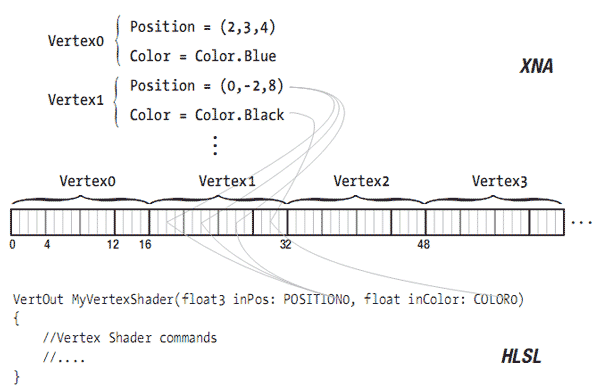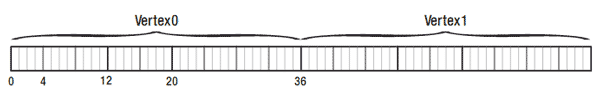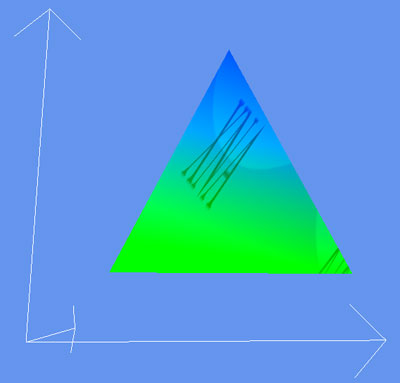# 5.12 创建自己的顶点格式

## 解决方案## 工作原理

### 重新创建VertexPositionColor结构

• 每个顶点自身的数据，为一个Vector3存储位置和一个Color
• 一个顶点的大小，这样显卡可以将数据流切割成单独的顶点
• VertexElements，它可以告知显卡每个顶点包含何种数据，显卡应该如何切割顶点以获得对应的数据。

#### 第1步

public struct MyVertexPositionColor
{
public Vector3 Position;
public Color Color;
public MyVertexPositionColor(Vector3 position, Color color)
{
Position = position;
Color = color;
}
}

#### 第2步

public static readonly int SizeInBytes = sizeof(float) * (3 + 1);

#### 第3步

public static readonly VertexElement[] VertexElements =
{
new VertexElement(0, 0, VertexElementFormat.Vector3, VertexElementMethod.Default,VertexElementUsage.Position, 0),
new VertexElement(0, sizeof(float)*3, VertexElementFormat.Color, VertexElementMethod.Default, VertexElementUsage.Color, 0),
}; 

### 完整的MyVertexPositionColor结构

public struct MyVertexPositionColor
{
public Vector3 Position;
public Color Color;
public MyVertexPositionColor(Vector3 position, Color color)
{
Position = position;
Color = color;
}

public static readonly VertexElement[] VertexElements =
{
new VertexElement(0, 0, VertexElementFormat.Vector3, VertexElementMethod.Default, VertexElementUsage.Position, 0),
new VertexElement(0, sizeof(float)*3, VertexElementFormat.Color, VertexElementMethod.Default, ~ VertexElementUsage.Color, 0),
};

public static readonly int SizeInBytes = sizeof(float) * (3 + 1);
}

### MyVertexPositionColor结构的用法

private void InitVertices()
{
myVertexDeclaration = new VertexDeclaration(device, MyVertexPositionColor.VertexElements);
MyVertexPositionColor[] vertices = new MyVertexPositionColor;

int i = 0;
vertices[i++] = new MyVertexPositionColor(new Vector3(1, 1, -1), Color.Red);
vertices[i++] = new MyVertexPositionColor(new Vector3(3, 5, -1), Color.Green);
vertices[i++] = new MyVertexPositionColor(new Vector3(5, 1, -1), Color.Blue);
vertBuffer = new VertexBuffer(device, MyVertexPositionColor.SizeInBytes * vertices.Length, BufferUsage.WriteOnly);
vertBuffer.SetData<MyVertexPositionColor>(vertices, 0, vertices.Length);
} 

basicEffect.Begin();
foreach (EffectPass pass in basicEffect.CurrentTechnique.Passes)
{
pass.Begin();
device.VertexDeclaration = myVertexDeclaration;
device.Vertices.SetSource(vertBuffer, 0, MyVertexPositionColor.SizeInBytes);
device.DrawPrimitives(PrimitiveType.TriangleList, 0, 1); pass.End();
}
basicEffect.End(); 

### 自定义顶点格式public struct MyCustomVertexFormat
{
public Vector3 Position;
public Vector2 TexCoords;
public Vector4 Extra;

public MyCustomVertexFormat(Vector3 Position, Vector2 TexCoords, Vector4 Extra)
{
this.Position = Position;
this.TexCoords = TexCoords;
this.Extra = Extra;
}

public static readonly VertexElement[] VertexElements =
{
new VertexElement(0, 0, VertexElementFormat.Vector3, VertexElementMethod.Default, VertexElementUsage.Position, 0),
new VertexElement(0, sizeof(float)*3, VertexElementFormat.Vector2, VertexElementMethod.Default, VertexElementUsage.TextureCoordinate, 0),
new VertexElement(0, sizeof(float)*(3+2), VertexElementFormat.Vector4, VertexElementMethod.Default, VertexElementUsage.TextureCoordinate, 1),
};

public static readonly int SizeInBytes = sizeof(float) * (3 + 2 + 4);
}

Vector4位于Position和Texture坐标数据之后，所以Vector4可以在字节sizeof(float)*(3+2) = 20处找到，可参见图5-25。

### 定义自定义格式的顶点

myVertexDeclaration = new VertexDeclaration(device, MyCustomVertexFormat.VertexElements);
MyCustomVertexFormat[] vertices = new MyCustomVertexFormat;

int i = 0;
vertices[i++] = new MyCustomVertexFormat(new Vector3(1, 1, -1), new Vector2(0,1), new Vector4(-1.0f,0.5f,0.3f,0.2f));
vertices[i++] = new MyCustomVertexFormat(new Vector3(3, 5, -1), new Vector2(0.5f, 0), new Vector4(0.8f, -0.2f, 0.5f, -0.2f));
vertices[i++] = new MyCustomVertexFormat(new Vector3(5, 1, -1), new Vector2(1, 1), new Vector4(2.0f, 0.6f, -1.0f, 0.4f));
vertBuffer = new VertexBuffer(device, MyCustomVertexFormat.SizeInBytes vertices.Length, ResourceUsage.WriteOnly);

vertBuffer.SetData<MyCustomVertexFormat>(vertices, 0, vertices.Length, SetDataOptions.None); 

// Technique: CustomVertexShader
CVVertexToPixel CVVertexShader(float3 inPos: POSITION0, float2 inTexCoord: TEXCOORD0, float4 inExtra: TEXCOORD1)
{
CVVertexToPixel Output = (CVVertexToPixel)0;
float4 origPos = float4(inPos, 1);
float4x4 preViewProjection = mul(xView, xProjection);
float4x4 preWorldViewProjection = mul(xWorld, preViewProjection);
Output.Position = mul(origPos, preWorldViewProjection);
Output.Extra = sin(xTime*inExtra.xyz);

Output.TexCoord = inTexCoord;
Output.TexCoord.x += sin(xTime)*inExtra.w;
Output.TexCoord.y -= inExtra.w;

return Output;
} 

CVPixelToFrame CVPixelShader(CVVertexToPixel PSIn) : COLOR0
{
CVPixelToFrame Output = (CVPixelToFrame)0;
Output.Color = tex2D(TextureSampler, PSIn.TexCoord);
Output.Color.rgb += PSIn.Extra.rgb; return Output;
}

effect.Parameters["xWorld"].SetValue(Matrix.Identity);
effect.Parameters["xView"].SetValue(fpsCam.ViewMatrix);
effect.Parameters["xProjection"].SetValue(fpsCam.ProjectionMatrix);
effect.Parameters["xTexture"].SetValue(texture);
effect.Parameters["xTime"].SetValue(time);

effect.Begin();
foreach (EffectPass pass in effect.CurrentTechnique.Passes)
{
pass.Begin();
device.VertexDeclaration = myVertexDeclaration;
device.Vertices.SetSource(vertBuffer, 0, MyCustomVertexFormat.SizeInBytes);
device.DrawPrimitives(PrimitiveType.TriangleList, 0, 1);
pass.End();
}
effect.End(); 

## 代码

XNA代码已经在前面写过了，下面的代码是HLSL文件的内容：

float4x4 xWorld;
float4x4 xView;
float4x4 xProjection;
float xTime;

Texture xTexture;
sampler TextureSampler = sampler_state
{
texture = <xTexture> ;
magfilter = LINEAR;
minfilter = LINEAR;
mipfilter=LINEAR;
};

struct CVVertexToPixel
{
float4 Position : POSITION;
float2 TexCoord : TEXCOORD0;
float3 Extra : TEXCOORD1;
};

struct CVPixelToFrame
{
float4 Color : COLOR0;
};

CVVertexToPixel CVVertexShader(float3 inPos: POSITION0, float2 inTexCoord: TEXCOORD0, float4 inExtra: TEXCOORD1)
{
CVVertexToPixel Output = (CVVertexToPixel)0;
float4 origPos = float4(inPos, 1);
float4x4 preViewProjection = mul(xView, xProjection);
float4x4 preWorldViewProjection = mul(xWorld, preViewProjection);

Output.Position = mul(origPos, preWorldViewProjection);
Output.Extra = sin(xTime*inExtra.xyz);
Output.TexCoord = inTexCoord;
Output.TexCoord.x += sin(xTime)*inExtra.w;
Output.TexCoord.y -= sin(xTime)*inExtra.w;

return Output;

}

{
CVPixelToFrame Output = (CVPixelToFrame)0;
Output.Color = tex2D(TextureSampler, PSIn.TexCoord);
Output.Color.rgb += PSIn.Extra.rgb;

return Output;
}

{
pass Pass0
{
}